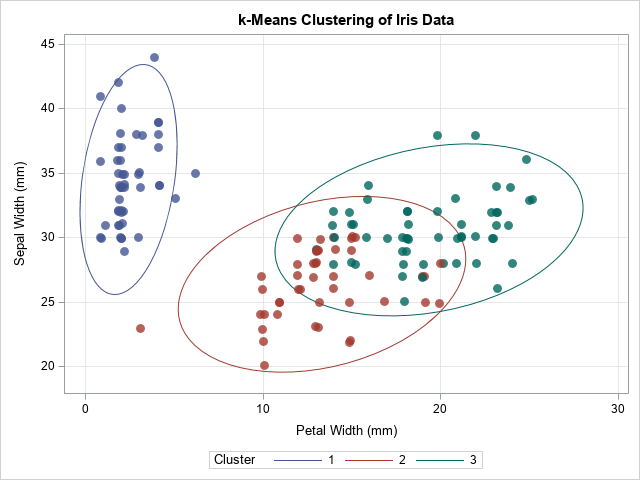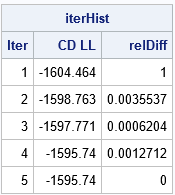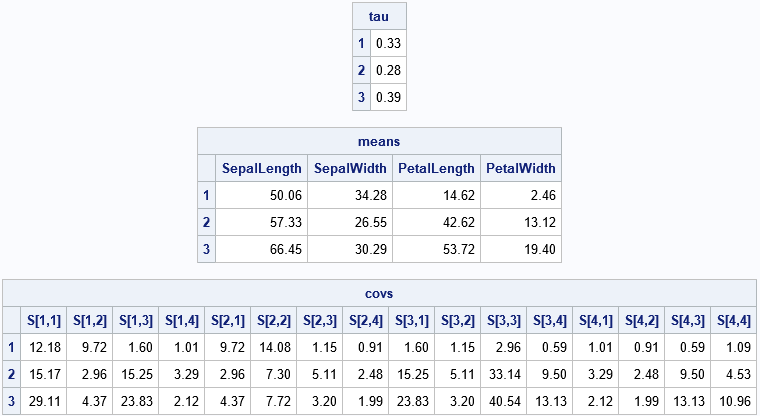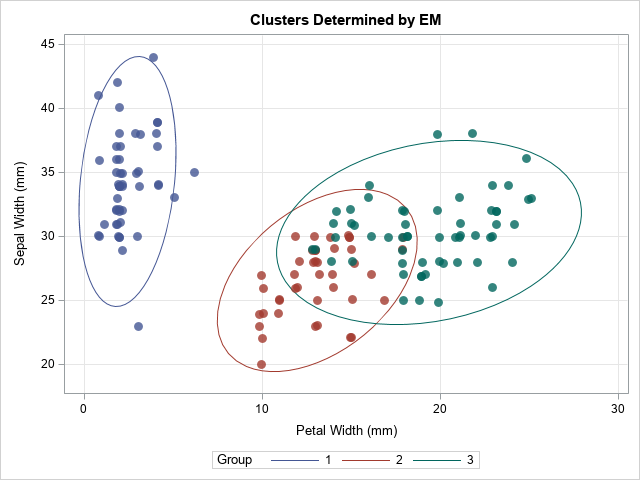Last month a SAS programmer asked how to fit a multivariate Gaussian mixture model in SAS. For univariate data, you can use the FMM Procedure, which fits a large variety of finite mixture models. If your company is using SAS Viya, you can use the MBC or GMM procedures, which perform model-based clustering (PROC MBC) or cluster analysis by using the Gaussian mixture model (PROC GMM). The MBC procedure is part of SAS Visual Statistics; The GMM procedure is part of SAS Visual Data Mining and Machine Learning (VDMML).

### The problem: Fitting a Gaussian mixture model

A Gaussian mixture model assumes that each cluster is multivariate normal but allows different clusters to have different within-cluster covariance structures. As in k-means clustering, it is assumed that you know the number of clusters, G. The clustering problem is an "unsupervised" machine learning problem, which means that the observations do not initially have labels that tell you which observation belongs to which cluster. The goal of the analysis is to assign each observation to a cluster ("hard clustering") or a probability of belonging to each cluster ("fuzzy clustering"). I will use the terms "cluster" and "group" interchangeably. In this article, I will only discuss the hard-clustering problem, which is conceptually easier to understand and implement.

The density of a Gaussian mixture with G components is $\Sigma_{i=1}^G \tau_i f(\mathbf{x}; {\boldsymbol\mu}_i, {\boldsymbol\Sigma}_i)$, where f(x; μi, Σi) is the multivariate normal density of the i_th component, which has mean vector μi and covariance matrix Σi. The values τi are the mixing probabilities, so Σ τi = 1. If x is a d-dimensional vector, you need to estimate τi, μi, and Σi for each of the G groups, for a total of G*(1 + d + d*(d-1)/2) – 1 parameter estimates. The d*(d-1)/2 expression is the number of free parameters in the symmetric covariance matrix and the -1 term reflects the constraint Σ τi = 1. Fortunately, the assumption that the groups are multivariate normal enables you to compute the maximum likelihood estimates directly without doing any numerical optimization.

Fitting a Gaussian mixture model is a "chicken-and-egg" problem because it consists of two subproblems, each of which is easy to solve if you know the answer to the other problem:

• If you know to which group each observation belongs, you can compute maximum likelihood estimates for the mean (center) and covariance of each group. You can also use the number of observations in each group to estimate the mixing probabilities for the finite mixture distribution.
• If you know the center, covariance matrix, and mixing probabilities for each of the G groups, you can use the density function for each group (weighted by the mixing probability) to determine how likely each observation is to belong to a cluster. For "hard clustering," you assign the observation to the cluster that gives the highest likelihood.

The expectation-maximization (EM) algorithm is an iterative method that enables you to solve interconnected problems like this. The steps of the EM algorithm are given in the documentation for the MBC procedure, as follows:

1. Use some method (such as k-means clustering) to assign each observation to a cluster. The assignment does not have to be precise because it will be refined. Some data scientists use random assignment as a quick-and-dirty way to initially assign points to clusters, but for hard clustering this can lead to less than optimal solutions.
2. The M Step: Assume that the current assignment to clusters is correct. Compute the maximum likelihood estimates of the within-cluster count, mean, and covariance. From the counts, estimate the mixing probabilities. I have previously shown how to compute the within-group parameter estimates
3. The E Step: Assume the within-cluster statistics are correct. I have previously shown how to evaluate the likelihood that each observation belongs to each cluster. Use the likelihoods to update the assignment to clusters. For hard clustering, this means choosing the cluster for which the density (weighted by the mixing probabilities) is greatest.
4. Evaluate the mixture log likelihood, which is an overall measure of the goodness of fit. If the log likelihood has barely changed from the previous iteration, assume that the EM algorithm has converged. Otherwise, go to the M step and repeat until convergence. The documentation for PROC MBC provides the log-likelihood function for both hard and fuzzy clustering.

This implementation of the EM algorithm performs the M-step before the E-step, but it is still the "EM" algorithm. (Why? Because there is no "ME" in statistics!)

### Use k-means clustering to assign the initial group membership

The first step is to assign each observation to a cluster. Let's use the Fisher Iris data, which we know has three clusters. We will not use the Species variable in any way, but merely treat the data as four-dimensional unlabeled data.

The following steps are adapted from the Getting Started example in PROC FASTCLUS. The call to PROC STDIZE standardizes the data and the call to PROC FASTCLUS performs k-means clustering and saves the clusters to an output data set.

/* standardize and use k-means clustering (k=3) for initial guess */ proc stdize data=Sashelp.Iris out=StdIris method=std; var Sepal: Petal:; run;   proc fastclus data=StdIris out=Clust maxclusters=3 maxiter=100 random=123; var Sepal: Petal:; run;   data Iris; merge Sashelp.Iris Clust(keep=Cluster); /* for consistency with the Species order, remap the cluster numbers */ if Cluster=1 then Cluster=2; else if Cluster=2 then Cluster=1; run;   title "k-Means Clustering of Iris Data"; proc sgplot data=Iris; ellipse x=PetalWidth y=SepalWidth / group=Cluster; scatter x=PetalWidth y=SepalWidth / group=Cluster transparency=0.2 markerattrs=(symbol=CircleFilled size=10) jitter; xaxis grid; yaxis grid; run;The graph shows the cluster assignments from PROC FASTCLUS. They are similar but not identical to the actual groups of the Species variable. In the next section, these cluster assignments are used to initialize the EM algorithm.

### The EM algorithm for hard clustering

You can write a SAS/IML program that implements the EM algorithm for hard clustering. The M step uses the MLEstMVN function (described in a previous article) to compute the maximum likelihood estimates within clusters. The E step uses the LogPdfMVN function (described in a previous article) to compute the log-PDF for each cluster (assuming MVN).

proc iml; load module=(LogPdfMVN MLEstMVN); /* you need to STORE these modules */   /* 1. Read data. Initialize 'Cluster' assignments from PROC FASTCLUS */ use Iris; varNames = {'SepalLength' 'SepalWidth' 'PetalLength' 'PetalWidth'}; read all var varNames into X; read all var {'Cluster'} into Group; /* from PROC FASTCLUS */ close;   nobs = nrow(X); d = ncol(X); G = ncol(unique(Group)); prevCDLL = -constant('BIG'); /* set to large negative number */ converged = 0; /* iterate until converged=1 */ eps = 1e-5; /* convergence criterion */ iterHist = j(100, 3, .); /* monitor EM iteration */ LL = J(nobs, G, 0); /* store the LL for each group */   /* EM algorithm: Solve the M and E subproblems until convergence */ do nIter = 1 to 100 while(^converged); /* 2. M Step: Given groups, find MLE for n, mean, and cov within each group */ L = MLEstMVN(X, Group); /* function returns a list */ ns = L$'n'; tau = ns / sum(ns); means = L$'mean'; covs = L\$'cov';   /* 3. E Step: Given MLE estimates, compute the LL for membership in each group. Use LL to update group membership. */ do k = 1 to G; LL[ ,k] = log(tau[k]) + LogPDFMVN(X, means[k,], shape(covs[k,],d)); end; Group = LL[ ,<:>]; /* predicted group has maximum LL */   /* 4. The complete data LL is the sum of log(tau[k]*PDF[,k]). For "hard" clustering, Z matrix is 0/1 indicator matrix. DESIGN function: https://blogs.sas.com/content/iml/2016/03/02/dummy-variables-sasiml.html */ Z = design(Group); /* get dummy variables for Group */ CDLL = sum(Z # LL); /* sum of LL weighted by group membership */ /* compute the relative change in CD LL. Has algorithm converged? */ relDiff = abs( (prevCDLL-CDLL) / prevCDLL ); converged = ( relDiff < eps );   /* monitor convergence; if no convergence, iterate */ prevCDLL = CDLL; iterHist[nIter,] = nIter || CDLL || relDiff; end;   /* remove unused rows and print EM iteration history */ iterHist = iterHist[ loc(iterHist[,2]^=.), ]; print iterHist[c={'Iter' 'CD LL' 'relDiff'}];The output from the iteration history shows that the EM algorithm converged in five iterations. At each step of the iteration, the log likelihood increased, which shows that the fit of the Gaussian mixture model improved at each iteration. This is one of the features of the EM algorithm: the likelihood always increases on successive steps.

### The results of the EM algorithm for fitting a Gaussian mixture model

This problem uses G=3 clusters and d=4 dimensions, so there are 3*(1 + 4 + 4*3/2) – 1 = 32 parameter estimates! Most of those parameters are the elements of the three symmetric 4 x 4 covariance matrices. The following statements print the estimates of the mixing probabilities, the mean vector, and the covariance matrices for each cluster. To save space, the covariance matrices are flattened into a 16-element row vector.

/* print final parameter estimates for Gaussian mixture */ GroupNames = strip(char(1:G)); rows = repeat(T(1:d), 1, d); cols = repeat(1:d, d, 1); SNames = compress('S[' + char(rows) + ',' + char(cols) + ']'); print tau[r=GroupNames F=6.2], means[r=GroupNames c=varNames F=6.2], covs[r=GroupNames c=(rowvec(SNames)) F=6.2];You can merge the final Group assignment with the data and create scatter plots that show the group assignment. One of the scatter plots is shown below:The EM algorithm changed the group assignments for 10 observations. The most obvious change is the outlying marker in the lower-left corner, which changed from Group=2 to Group=1. The other markers that changed are near the overlapping region between Group=2 and Group=3.

### Summary

This article shows an implementation of the EM algorithm for fitting a Gaussian mixture model. For simplicity, I implemented an algorithm that uses hard clustering (the complete data likelihood model). This algorithm might not perform well with a random initial assignment of clusters, so I used the results of k-means clustering (PROC FASTCLUS) to initialize the algorithm. Hopefully, some of the tricks and techniques in this implementation of the EM algorithm will be useful to SAS programmers who want to write more sophisticated EM programs.

The main purpose of this article is to demonstrate how to implement the EM algorithm in SAS/IML. As a reminder, if you want to fit a Gaussian mixture model in SAS, you can use PROC MBC or PROC GMM in SAS Viya. The MBC procedure is especially powerful: in Visual Statistics 8.5 you can choose from 17 different ways to model the covariance structure in the clusters. The MBC procedure also supports several ways to initialize the group assignments and supports both hard and fuzzy clustering. For more about the MBC procedure, see Kessler (2019), "Introducing the MBC Procedure for Model-Based Clustering."

I learned a lot by writing this program, so I hope it is helpful. You can download the complete SAS program that implements the EM algorithm for fitting a Gaussian mixture model. Leave a comment if you find it useful.